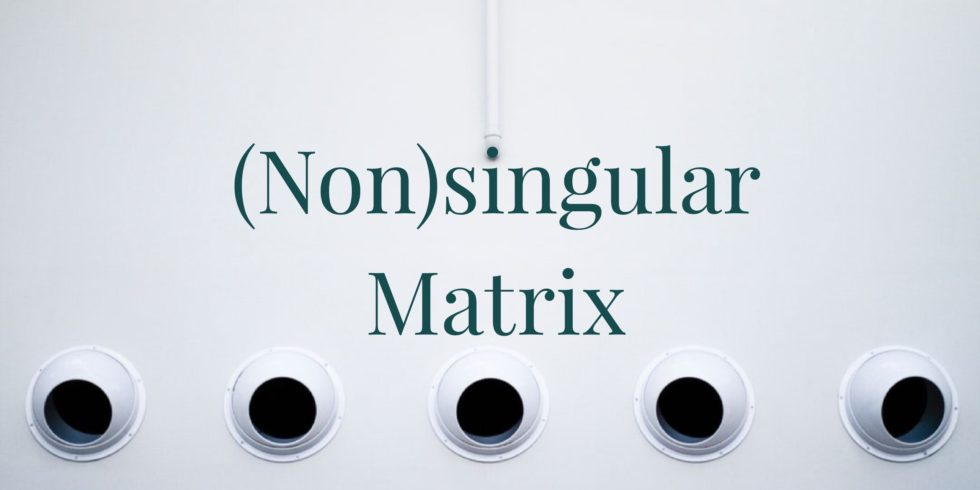# Determine whether the Given 3 by 3 Matrices are Nonsingular## Problem 671

Determine whether the following matrices are nonsingular or not.

(a) $A=\begin{bmatrix} 1 & 0 & 1 \\ 2 &1 &2 \\ 1 & 0 & -1 \end{bmatrix}$.

(b) $B=\begin{bmatrix} 2 & 1 & 2 \\ 1 &0 &1 \\ 4 & 1 & 4 \end{bmatrix}$.Add to solve later

## Solution.

Recall that an $n\times n$ matrix is nonsingular if $A\mathbf{x}=\mathbf{0}$ has only the zero solution. This is equivalent to the condition that the rank of $A$ is $n$.

### (a) Is $A=\begin{bmatrix} 1 & 0 & 1 \\ 2 &1 &2 \\ 1 & 0 & -1 \end{bmatrix}$ nonsingular?

We find the rank of the matrix $A$ as follows.
\begin{align*}
A=\begin{bmatrix}
1 & 0 & 1 \\
2 &1 &2 \\
1 & 0 & -1
\end{bmatrix}
\xrightarrow[R_3-R_1]{R_2-2R_1} \begin{bmatrix}
1 & 0 & 1 \\
0 &1 &0 \\
0 & 0 & -2
\end{bmatrix}
\xrightarrow{-\frac{1}{2}R_3}
\begin{bmatrix}
1 & 0 & 1 \\
0 &1 &0 \\
0 & 0 & 1
\end{bmatrix}
\xrightarrow{R_1-R_3}
\begin{bmatrix}
1 & 0 & 0 \\
0 &1 &0 \\
0 & 0 & 1
\end{bmatrix}.
\end{align*}
The last matrix is in reduced row echelon form and has three nonzero rows.
Hence, the rank of $A$ is $3$.
It follows that the matrix $A$ is nonsingular.

### (b) Is $B=\begin{bmatrix} 2 & 1 & 2 \\ 1 &0 &1 \\ 4 & 1 & 4 \end{bmatrix}$ nonsingular?

Next, we compute the rank of $B$ as follows.
\begin{align*}
B=\begin{bmatrix}
2 & 1 & 2 \\
1 &0 &1 \\
4 & 1 & 4
\end{bmatrix}
\xrightarrow{R_1 \leftrightarrow R_2}
\begin{bmatrix}
1 & 0 & 1 \\
2 &1 &2 \\
4 & 1 & 4
\end{bmatrix}
\xrightarrow[R_3-4R_1]{R_2-2R_1}
\begin{bmatrix}
1 & 0 & 1 \\
0 &1 &0 \\
0 & 1 & 0
\end{bmatrix}
\xrightarrow{R_3-R_2}
\begin{bmatrix}
1 & 0 & 1 \\
0 &1 &0 \\
0 & 0 & 0
\end{bmatrix}.
\end{align*}

This computation shows that the rank of $B$ is $2$.
Hence, the matrix $B$ is singular.Add to solve later

### More from my site

#### You may also like...

This site uses Akismet to reduce spam. Learn how your comment data is processed.

###### More in Linear Algebra##### For What Values of $a$, Is the Matrix Nonsingular?

Determine the values of a real number $a$ such that the matrix \[A=\begin{bmatrix} 3 & 0 & a \\ 2...

Close